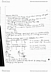# ECON 1000 Lecture Notes - Inferior Good, Normal Good

72 views3 pages
School
Department
Coursecastroariane563 and 39059 others unlocked10
Verified Note
10 documents

## Document Summary

A measure of the responsiveness of the quantity demanded to a price change. The price elasticity of demand is a units-free measure of the responsiveness of the quantity demanded of a good to a change in its price when all other influences on buyers" plans remain the same. The price elasticity of demand is calculated by using the formula: Percentage change in price if =1, unit elastic demand. (new quantity- original quantity) /quantity average (new price original price)/ price average. If the quantity demanded doesn"t change when the price changes, the price elasticity of demand is zero and the good as a perfectly inelastic demand. if <1, inelastic demand if >1, elastic demand. The total revenue price * demand (when the price changes, total revenue also changes. But a rise in price doesn"t always increase total revenue. ) The change in total revenue due to a change in price depends on the elasticity of demand: